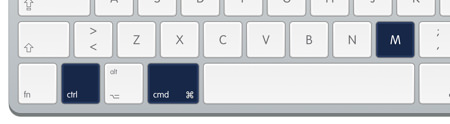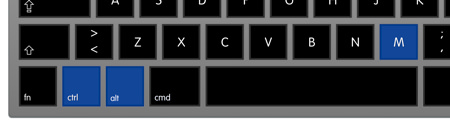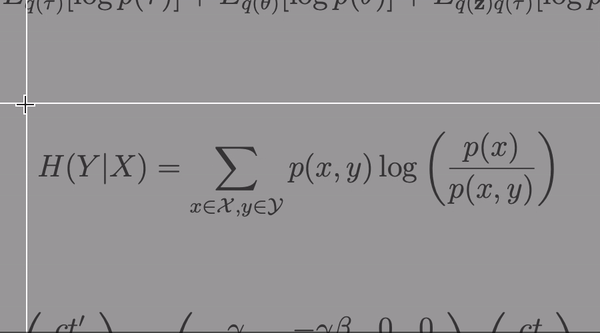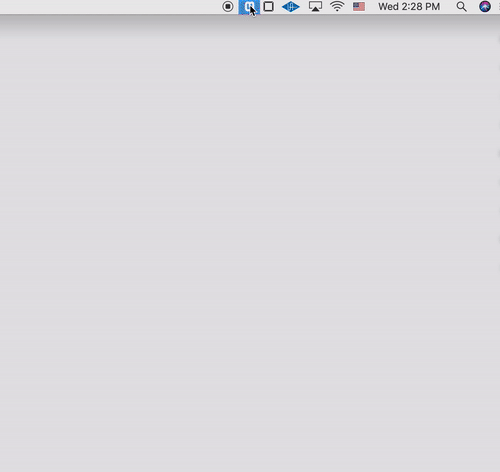# Convert images to LaTeX

Take a screenshot of math and paste the LaTeX into your editor, all with a single keyboard shortcut.

• “If I had known about Mathpix earlier, perhaps I would have had enough time to work out the Grand Unified Theory.”

#### Albert Einstein

• “When I lost my .tex file to the Principia, I was devastated. Mathpix helped me effortlessly use equations from the Principia in my new work. I now have more time to stand beneath trees and get hit by apples.”

#### Isaac Newton

• “Mathpix's AI definitely passes this Turing test!”

• 1

## Start

by entering the keyboard shortcut

Ctrl + ⌘ + M on MacCtrl + Alt + M on Windows & Linux• 2

## Capture

your desired inputs within the screenshot box

Just click and drag• 3

## Edit• 4

## Paste

the LaTeX into any compatible editor

## Recognition features

\Gamma _ { \epsilon } ( x ) = [ 1- e ^ { - 2\pi \epsilon } ] ^ { 1- x } \prod _ { n = 0} ^ { \infty } \frac { 1- \operatorname{exp} ( - 2\pi \epsilon ( n + 1) ) } { 1- \operatorname{exp} ( - 2\pi \epsilon ( x + n ) ) }

\left( \begin{array} c t ^ { \prime } \\ x ^ { \prime } \\ y ^ { \prime } \\ z ^ { \prime } \end{array} \right) = \left( \begin{array} { c c c c } { \gamma } & { - \gamma \beta } & { 0 } & { 0 } \\ { - \gamma \beta } & { \gamma } & { 0 } & { 0 } \\ { 0 } & { 0 } & { 1 } & { 0 } \\ { 0 } & { 0 } & { 0 } & { 1 } \end{array} \right) \left( \begin{array} c t \\ x \\ y \\ z \end{array} \right)

6 \mathrm { CO } _ { 2 } + 6 \mathrm { H } _ { 2 } \mathrm { O } \rightarrow \mathrm { C } _ { 6 } \mathrm { H } _ { 12 } \mathrm { O } _ { 6 } + 6 \mathrm { O } _ { 2 }

We can also read large chunks of text that contain multiple lines. This is really helpful for when you want to extract a chunk of text from a PDF because you are unable to copy the source text directly.

Snip fits into your workflow with:

• Typora

• StackEdit

• MacDown

• Authorea

• TeXstudio

• TeXworks

• TeXmaker

• Overleaf

and any other editors that accept LaTeX